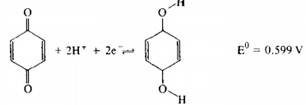### Create an Account

Home / Questions / Quinone undergoes a reversible reduction at a voltammetric working electrode The reaction ...

# Quinone undergoes a reversible reduction at a voltammetric working electrode The reaction is a Assume that the diffusion coefficient for quinone and hydroquinone are approximately the same and

Quinone undergoes a reversible reduction at a voltammetric working electrode. The reaction is(a) Assume that the diffusion coefficient for quinone and hydroquinone are approximately the same and calculate the approximate half-wave potential (versus SCE) for the reduction of hydroquinone at an ROE from a solution buffered to a pH of 7.0.

(b) Repeat the calculation in (a) for a solution buffered to a pH of 5.0.

Jun 23 2020 View more View LessSubscribe To Get Solution